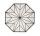Triangle

Calculate the area of right triangle ΔABC, if one leg is long 14 and its opposite angle is 59°.

Result

S =  58.9

Solution:Leave us a comment of example and its solution (i.e. if it is still somewhat unclear...):Be the first to comment!To solve this example are needed these knowledge from mathematics:

See also our right triangle calculator. Most natural application of trigonometry and trigonometric functions is a calculation of the triangles. Common and less common calculations of different types of triangles offers our triangle calculator. Word trigonometry comes from Greek and literally means triangle calculation.

Next similar examples:

1. Right triangle ABCCalculate the perimeter and area of a right triangle ABC, if you know the length of legs 4 cm 5.5 cm and 6.8 cm is hypotenuse.
2. Chord 2Point A has distance 13 cm from the center of the circle with radius r = 5 cm. Calculate the length of the chord connecting the points T1 and T2 of contact of tangents led from point A to the circle.
3. Right triangleRight triangle legs has lengths 630 mm and 411 dm. Calculate the area of this triangle.
4. Right triangleRight triangle ABC with side a = 19 and the area S = 95. Calculate the length of the remaining sides.
5. HypotenuseCalculate the length of the hypotenuse of a right triangle if the length of one leg is 4 cm and its content area is 16 square centimeters.
6. Four ropesTV transmitter is anchored at a height of 44 meters by four ropes. Each rope is attached at a distance of 55 meters from the heel of the TV transmitter. Calculate how many meters of rope were used in the construction of the transmitter. At each attachment.
7. Height 2Calculate the height of the equilateral triangle with side 38.
8. CablewayCableway has a length of 1800 m. The horizontal distance between the upper and lower cable car station is 1600 m. Calculate how much meters altitude is higher upper station than the base station.
9. Parallelogram +ľ| AB | = 76cm, | BC | = 44cm, angle BAD = 30 °. Find the area of the parallelogram.
10. Octagonal matOctagonal mat formed from a square plate with a side of 40 cm so that every corner cut the isosceles triangle with leg 3.6 cm. What is the content area of one mat?
11. HeightIs right that in any right triangle height is less or equal half of the hypotenuse?
12. Center traverseIt is true that the middle traverse bisects the triangle?
13. AceThe length of segment AB is 24 cm and the point M and N divided it into thirds. Calculate the circumference and area of this shape.
14. Circular lawnAround a circular lawn area is 2 m wide sidewalk. The outer edge of the sidewalk is curb whose width is 2 m. Curbstone and the inner side of the sidewalk together form a concentric circles. Calculate the area of the circular lawn and the result round to 1
15. Trapezium 2Trapezium has an area of 24 square cms. How many different trapeziums can be formed ?
16. PercentsHow many percents is 900 greater than the number 750?
17. Linear systemSolve this linear system (two linear equations with two unknowns): x+y =36 19x+22y=720